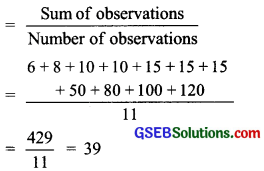# GSEB Solutions Class 7 Maths Chapter 3 Data Handling Ex 3.2

Gujarat Board GSEB Textbook Solutions Class 7 Maths Chapter 3 Data Handling Ex 3.2 Textbook Questions and Answers.

## Gujarat Board Textbook Solutions Class 7 Maths Chapter 3 Data Handling Ex 3.2Question 1.
The scores in mathematics test (out of 25) of 15 students is as follows:
19, 25, 23, 20, 9, 20, 15, 10, 5, 16, 25, 20, 24, 12, 20
Find the mode and median of this data. Are they same?
Solution:
Writing the data in ascending order, we have:
5, 9, 10, 12, 15, 16, 19, 20, 20, 20, 20, 23, 24, 25, 25
∵ Highest occurring observation is 20.
∴ Mode of the data = 20
Again, the middle value = 20
∴ Median of the data = 20
Obviously, here the mode and median are the same.

Question 2.
The runs scored in a cricket match by 11 players is as follows:
6, 15, 120, 50, 100, 80, 10, 15, 8, 10, 15
Find the mean, mode and median of this data. Are the three same?
Solution:
Arranging the given data in an ascending order, we have:
6, 8, 10, 10, 15, 15, 15, 50, 80, 100, 120
(i) Mean of the data(ii) ∵ Highest occurring observation is 15.
∴ Mode of the data = 15.

(iii) ∵ The value of the middle observation is 15.
∴ Median of the data is 15.
Here, mean, mode and median are not the same.Question 3.
The weights (in kg) of 15 students of a class are:
38, 42, 35, 37, 45, 50, 32, 43, 43, 40, 36, 38, 43, 38, 47
(i) Find the mode and median of this data.
(ii) Is there more than one model?
Solution:
Arranging the given data in ascending order, we have:
32, 35, 36, 37, 38, 38, 38, 40, 42, 43, 43, 43, 45, 47, 50

(i) Mode:
∵ The most occurring observations are 38, and 43.
∴ Modes of the data are 38 and 43.

Median:
∵ Value of the middle term is 40.
∴ Median of the data is 40.

(ii) Yes, there are more than one mode.

Question 4.
Find the mode and median of the data:
13, 16, 12, 14, 19, 12, 14, 13, 14
Solution:
Writing the given data in ascending order, we have:
12, 12, 13, 13, 14, 14, 14, 16, 19
∵ Highest occurring observation is 14.
∴ Mode of the given data =14
Again, the value of the middle observation is 14.
∴ Median of the data = 14.Question 5.
Tell whether the statement is true or false:
(i) The mode is always one of the numbers in a data.
(ii) The mean is one of the numbers in a data.
(iii) The median is always one of the numbers in a data.
(iv) The data 6, 4, 3, 8, 9, 12, 13, 9 has mean 9.
Solution:
(i) True
(ii) False
(iii) True
(iv) False